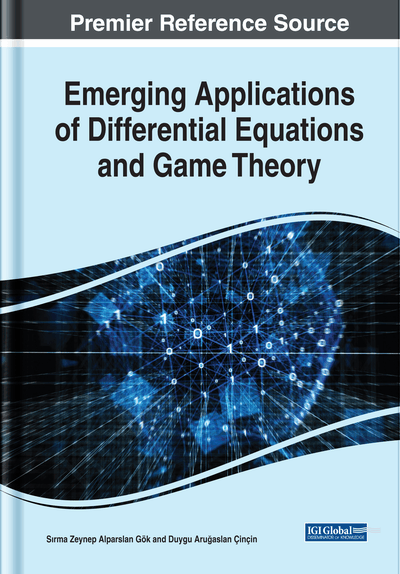Stability Analysis of a Nonlinear Epidemic Model With Generalized Piecewise Constant Argument

Duygu Aruğaslan-Çinçin (Süleyman Demirel University, Turkey) and Nur Cengiz (Süleyman Demirel University, Turkey)
DOI: 10.4018/978-1-7998-0134-4.ch009
Available
\$37.50
No Current Special Offers

Abstract

The authors consider a nonlinear epidemic equation by modeling it with generalized piecewise constant argument (GPCA). The authors investigate invariance region for the considered model. Sufficient conditions guaranteeing the existence and uniqueness of the solutions of the model are given by creating integral equations. An important auxiliary result giving a relation between the values of the unknown function solutions at the deviation argument and at any time t is indicated. By using Lyapunov-Razumikhin method developed by Akhmet and Aruğaslan for the differential equations with generalized piecewise constant argument (EPCAG), the stability of the trivial equilibrium is investigated in addition to the stability examination of the positive equilibrium transformed into the trivial equilibrium. Then sufficient conditions for the uniform stability and the uniform asymptotic stability of trivial equilibrium and the positive equilibrium are given.
Chapter Preview
Top

Background

Differential equations are very valuable in understanding the real life problems since they allow the mathematical expression of the real phenomena. However, modeling of problems with ordinary differential equations is often not enough. Because, while problems are set up mathematically by neglecting the discontinuous effects, the models and thus the results of their qualitative analysis are far distance from the reality. This necessitated the introduction and development of the theory of differential equations with discontinuities. One type of equation developed as a result of this requirement is the differential equations with deviating arguments. Differential equations with deviating arguments host many classes of equations, such as functional differential equations, differential equations with delay, piecewise constant argument and generalized piecewise constant argument. Differential equations in which the highest order derivative of the unknown function depends on the previous values of the unknown function are called delay differential equations. A delay differential equation is most fundamentally given by

Here, τ is the positive constant specified in the amount of delay, and it can be seen that the development of the state vector depends on the current time, the value of the state vector at this moment and also on the previous values of the process.

In the early 1980s, differential equations with piecewise constant argument which are in the class of delay differential equations are defined by Cooke and Wiener (1984) in the form of

The literature knowledge is intense for studies based on the transformation of such equations into discrete equations. While models are established by differential equations with piecewise constant argument, change between the arguments is constant and always one unit since the greatest integer function is taken as the deviation argument. However, this approach can contradict with the reality phenomenon. Because it is important to approach the fact of reality when establishing the real life problems. In the light of this idea, the theory of differential equations with deviating arguments have been developed over time. Differential equations with piecewise constant argument are generalized by Akhmet (2007a, 2007b) by taking an arbitrary piecewise constant function instead of the greatest integer function as deviation argument. In this way, differential equations with GPCA are given by the following equations

Key Terms in this Chapter

SIS (Susceptible-Infected-Susceptible): It is a simple mathematical model of epidemics.

GPCA: Generalized piecewise constant argument is a deviation argument which is a piecewise function considered in differential equations with piecewise constant argument of generalized type.

Outbreak: Sometimes distinguished from an epidemic as more localized, or the term less likely to evoke public panic.

Population Dynamics: Population dynamics is the branch of life sciences that studies the size and age composition of populations as dynamical systems, and the biological and environmental processes driving them (such as birth and death rates, and by immigration and emigration). Example scenarios are ageing populations, population growth, or population decline.

Epidemic: The occurrence of more cases of disease, injury or other health condition than expected in a given area or among a specific group of persons during a particular period. The cases are usually presumed to have a common cause or to be related to one another in some way.

Complete Chapter List

Search this Book:
Reset